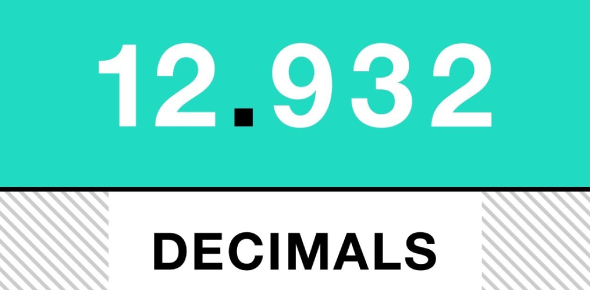# Operations With Decimals! Math Trivia Quiz

30 Questions | Attempts: 663
ShareSettingsCan you pass this Operations with Decimals in Math Quiz? Decimals numbers are based on the number ten, and as one moves to the left of a decimal number, each position is ten times more prominent, and the verse versa is true. Operations with decimals can be challenging if one does not know the rules associated with using the four functions on decimals. This quiz is designed to see how skilled you are at handling suck math problems. Give it a try!

• 1.
56.7 − 3.56 = [Blank]
• 2.
54.66 + 40.9 = [Blank]
• 3.
2.61 − 1.3 = [Blank]
• 4.
9.69 + 7.02 = [Blank]
• 5.
53.4 − 7.54 = [Blank]
• 6.
32.68 + 13.9 = [Blank]
• 7.
73.8 − 3.36 =  [Blank]
• 8.
34.2 + 1.48 = [Blank]
• 9.
44.84 − 44.8 = [Blank]
• 10.
67.4 + 44.9 = [Blank]
• 11.
3.94 − 3.1 = [Blank]
• 12.
49.7 + 4.3 = [Blank]
• 13.
19.47 − 8.4 = [Blank]
• 14.
48.3 + 8.6 = [Blank]
• 15.
70.2 + 9.62 = [Blank]
• 16.
45.86 − 23.67 = [Blank]
• 17.
51.18 + 3.92 = [Blank]
• 18.
4.7 − 3.5 = [Blank]
• 19.
85.4 + 3.70 = [Blank]
• 20.
27.07 − 18.22 = [Blank]
• 21.
1.7 x 3 = [Blank]
• 22.
1.5 x 4 = [Blank]
• 23.
3.3 x 9 = [Blank]
• 24.
8.4 x 2 = [Blank]
• 25.
5.4 x 4 = [Blank]

## Related TopicsBack to top
×

Wait!
Here's an interesting quiz for you.